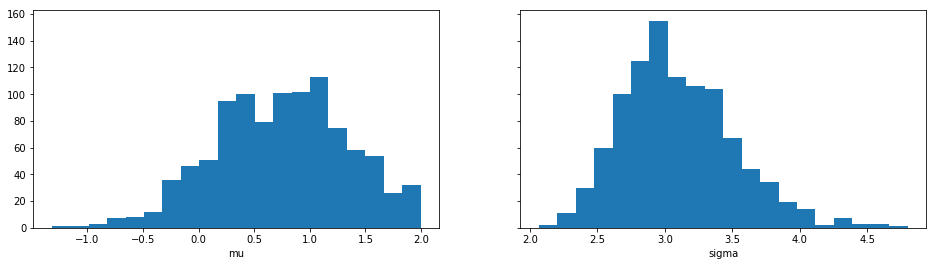# Quickstart

First ensure you have installed Python 3.7 (or greater) and ELFI. After installation you can start using ELFI:

```import elfi
```

ELFI includes an easy to use generative modeling syntax, where the generative model is specified as a directed acyclic graph (DAG). Let’s create two prior nodes:

```mu = elfi.Prior('uniform', -2, 4)
sigma = elfi.Prior('uniform', 1, 4)
```

The above would create two prior nodes, a uniform distribution from -2 to 2 for the mean `mu` and another uniform distribution from 1 to 5 for the standard deviation `sigma`. All distributions from `scipy.stats` are available.

For likelihood-free models we typically need to define a simulator and summary statistics for the data. As an example, lets define the simulator as 30 draws from a Gaussian distribution with a given mean and standard deviation. Let’s use mean and variance as our summaries:

```import scipy.stats as ss
import numpy as np

def simulator(mu, sigma, batch_size=1, random_state=None):
mu, sigma = np.atleast_1d(mu, sigma)
return ss.norm.rvs(mu[:, None], sigma[:, None], size=(batch_size, 30), random_state=random_state)

def mean(y):
return np.mean(y, axis=1)

def var(y):
return np.var(y, axis=1)
```

Let’s now assume we have some observed data `y0` (here we just create some with the simulator):

```# Set the generating parameters that we will try to infer
mean0 = 1
std0 = 3

# Generate some data (using a fixed seed here)
np.random.seed(20170525)
y0 = simulator(mean0, std0)
print(y0)
```
```[[ 3.7990926   1.49411834  0.90999905  2.46088006 -0.10696721  0.80490023
0.7413415  -5.07258261  0.89397268  3.55462229  0.45888389 -3.31930036
-0.55378741  3.00865492  1.59394854 -3.37065996  5.03883749 -2.73279084
6.10128027  5.09388631  1.90079255 -1.7161259   3.86821266  0.4963219
1.64594033 -2.51620566 -0.83601666  2.68225112  2.75598375 -6.02538356]]
```

Now we have all the components needed. Let’s complete our model by adding the simulator, the observed data, summaries and a distance to our model:

```# Add the simulator node and observed data to the model
sim = elfi.Simulator(simulator, mu, sigma, observed=y0)

# Add summary statistics to the model
S1 = elfi.Summary(mean, sim)
S2 = elfi.Summary(var, sim)

# Specify distance as euclidean between summary vectors (S1, S2) from simulated and
# observed data
d = elfi.Distance('euclidean', S1, S2)
```

If you have `graphviz` installed to your system, you can also visualize the model:

```# Plot the complete model (requires graphviz)
elfi.draw(d)
```Note

The automatic naming of nodes may not work in all environments e.g. in interactive Python shells. You can alternatively provide a name argument for the nodes, e.g. `S1 = elfi.Summary(mean, sim, name='S1')`.

We can try to infer the true generating parameters `mean0` and `std0` above with any of ELFI’s inference methods. Let’s use ABC Rejection sampling and sample 1000 samples from the approximate posterior using threshold value 0.5:

```rej = elfi.Rejection(d, batch_size=10000, seed=30052017)
res = rej.sample(1000, threshold=.5)
print(res)
```
```Method: Rejection
Number of samples: 1000
Number of simulations: 120000
Threshold: 0.492
Sample means: mu: 0.748, sigma: 3.1
```

Let’s plot also the marginal distributions for the parameters:

```import matplotlib.pyplot as plt
res.plot_marginals()
plt.show()
```